## Geodesic Dome

A Triangulation of a Platonic Solid or other Polyhedron to produce a close approximation to a Sphere. Theth order geodesation operation replaces each polygon of the polyhedron by the projection onto the Circumsphere of the orderregular tessellation of that polygon. The above figure shows geodesations of orders 1 to 3 (from top to bottom) of the Tetrahedron, Cube, Octahedron, Dodecahedron, and Icosahedron (from left to right).

R. Buckminster Fuller designed the first geodesic dome (i.e., geodesation of a Hemisphere). Fuller's dome was constructed from an Icosahedron by adding Isosceles Triangles about each Vertex and slightly repositioning the Vertices. In such domes, neither the Vertices nor the centers of faces necessarily lie at exactly the same distances from the center. However, these conditions are approximately satisfied.

In the geodesic domes discussed by Kniffen (1994), the sum of Vertex angles is chosen to be a constant. Given a Platonic Solid, let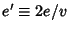be the number of Edges meeting at a Vertex andbe the number of Edges of the constituent Polygon. Call the angle of the old Vertex pointand the angle of the new Vertex point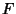. Then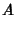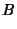(1)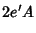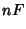(2)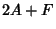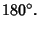(3)

Solving forgives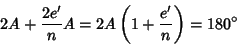(4)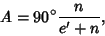(5)

and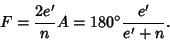(6)

The Vertex sum is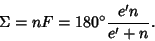(7)

 Solid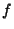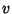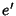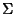Tetrahedron 3 3 45° 90° 270° Cube 24 14 3 4 51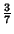° 81° 308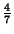° Octahedron 4 3 38° 108° 308° Dodecahedron 60 32 3 5 56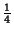° 71° 337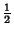° Icosahedron 5 3 33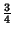° 118° 337°

References

Kenner, H. Geodesic Math and How to Use It. Berkeley, CA: University of California Press, 1976.

Kniffen, D. Geodesic Domes for Amateur Astronomers.'' Sky and Telescope, pp. 90-94, Oct. 1994.

Pappas, T. Geodesic Dome of Leonardo da Vinci.'' The Joy of Mathematics. San Carlos, CA: Wide World Publ./Tetra, p. 81, 1989.